Courses

# Chapter 7 - Frequency Domain Analysis(Part - 1) - Notes, Control System, Electrical Engineering Electrical Engineering (EE) Notes | EduRev

## Electrical Engineering (EE) : Chapter 7 - Frequency Domain Analysis(Part - 1) - Notes, Control System, Electrical Engineering Electrical Engineering (EE) Notes | EduRev

The document Chapter 7 - Frequency Domain Analysis(Part - 1) - Notes, Control System, Electrical Engineering Electrical Engineering (EE) Notes | EduRev is a part of the Electrical Engineering (EE) Course Electrical Engineering SSC JE (Technical).
All you need of Electrical Engineering (EE) at this link: Electrical Engineering (EE)

• Consider a linear system with a sinusoidal input r(t) = A sinωt under steady-state, the system outputs as well as the signals at all other points in the system are sinusoidal.
• The steady-state output may be written as c(t) = B sin (ωt + Ø)
• The magnitude and phase relationship between the sinusoidal input and the steady-state output of a system is termed the frequency response.
• In the LTI system, the frequency response is independent of the amplitude and phase of the input signal.
• For systems with very large time constants, the frequency response test is cumbersome to perform as the time required for the outputs to reach a steady-state for each frequency of the test signal is excessively long. Therefore, the frequency response test is not recommended for systems with very large time constants.
• The design and parameter adjustment of the open-loop transfer function of a system for specified closed-loop performance is carried out somewhat more easily in the frequency domain than in the time domain.
• Further the effects of noise disturbance and parameter variation are relatively easy to visualize and assess through frequency response. If necessary the transient response of a system can be obtained from its frequency response through the Fourier integral.
• An interesting and revealing comparison f frequency and time domain approaches is based on the relative stability studies of feedback systems.
• The Routh criterion is a time-domain approach that establishes with relative ease the stability of a system, but its adoption to determine the relative stability is involved and requires repeated application of the criterion. The roots locus method is a very powerful time-domain approach as it reveals not only stability but also the actual time response of the system. On the other hand, the Nyquist Criterion (discussed later) is a powerful frequency-domain method of extracting information regarding stability as well as the relative stability of a system without the need to evaluate the roots of the characteristic equation.
• The frequency response is easily evaluated from the sinusoidal transfer function which can be obtained simply by replacing s with jω in the system transfer function T(s). The transfer function T(jω) thus obtained, is a complex function of frequency and has both the magnitude and the phase angle. These characteristics are conveniently represented by graphical plots.
Correlation between Time and Frequency Response
As discussed earlier, the correlation between time and frequency response has an explicit form only for systems of first and second-order. Let us first discuss the explicit correlation for second-order systems.

Second order-Systems

• A second-order system of the form shown in the figure has the transfer function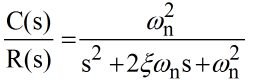where ξ is the damping factor and ωn is the undamped natural frequency of oscillations.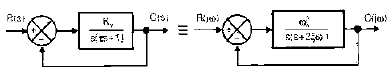•  The sinusoidal transfer function of the system is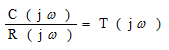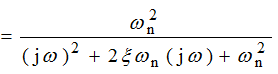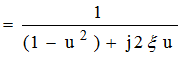where, u = ω/ωn is the normalized driving signal frequency From equation (8.1) we get T( jω )| = M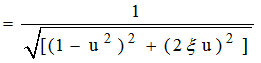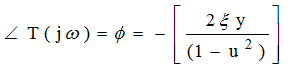• The steady-state output of the system for a sinusoidal input of unit magnitude and variable frequency ω is given by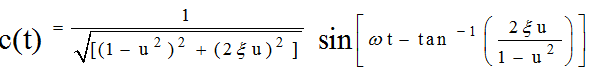• From equations (8.2) and (8.3), It is seen that when

u = 0,       m = 1          and Ø = 0

u = 1,      M = 1/2ξ      and Ø = π/2

u → ∞,    M → 0         and Ø = -π

• The magnitude and phase angle characteristic for normalized frequency 'u' for certain values of θ are shown below in figure (a) and (b) respectively. 
• The frequency where M has a peak value is known as the resonant frequency. At this frequency, the slope of the magnitude curve is zero Let ω,  be the resonant frequency and ur = (ur, un) be the normalized resonant frequency.  Then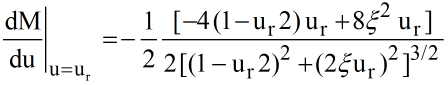= 0

Which gives,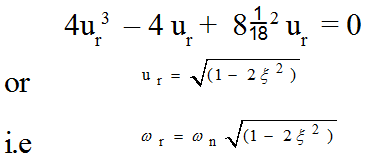• From equation (8.2), the maximum value of the magnitude known as the resonant peak is given by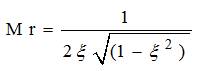•  The phase angle Ø of T (jω) at the resonant frequency obtained from equation (8.3) is given by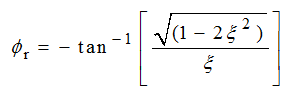From equations (8.4) and (8.5). it is seen that as x approaches zero, ωr approaches ωn and Mr approaches infinity. For ξ ≤ 1 /2, the resonant frequency always has a value less than ωn and the resonant peak has a value greater than 1.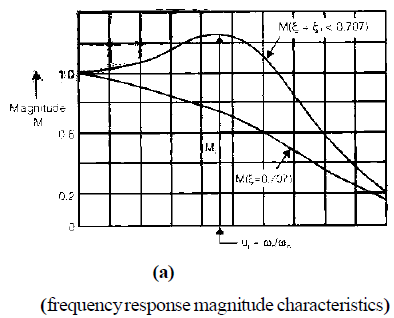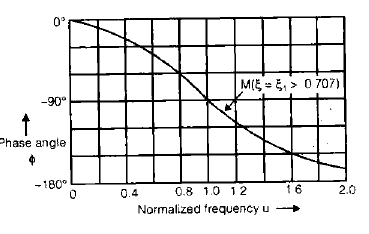Frequency response phase characteristic

• For ξ >1/√2, it is seen from equation (8.4) that dM/du, the slope of the magnitude curve does not become zero for any real value of ω. For this range of x, the magnitude M decreases monotonically from M = 1 at u = 0 with increasing u, as shown in the figure, it, therefore, follows that for ξ >1/√2, there is no resonant peak as such as the greatest value of M equal one. 
• The resonant frequency 1/16r of the frequency response is indicative of its natural frequency for a given1/18 and hence indicative of its speed of response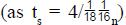. Mr and 1/16of the frequency response could thus be used as performance indices for the second-order system.
• Re-examining the figure, we notice that for ω > ωr, M decreases monotonically. The frequency at which M has a value of 1 / √2 is of special significance and is called the cut-off frequency wc. The signal frequencies above the cut-off are greatly attenuated in passing through a system.
• For feedback control systems, the range of frequencies over which M is equal to or greater than 1 /√2 is defined as bandwidth ωb. Control system being low pass filters (at zero frequency, M = 1), the bandwidth wb is equal to cut-off frequency ωc. The definition of the bandwidths depicted on the typical frequency response of the feedback control system is given in the figure below.
• In general, the bandwidth of a control system indicates the noise-filtering characteristics of the system. Also, bandwidth gives a measure of the transient response properties as observed below.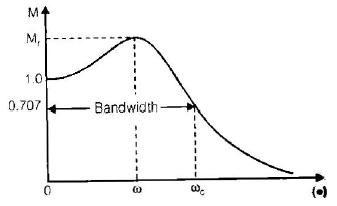The normalized bandwidth ub = ωbn of the second-order system under consideration can be readily determined as follows: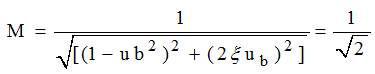or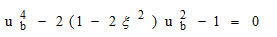Solving for ub we get,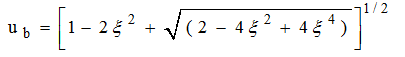• We observe from equation (8.6) that the normalized bandwidth is a function of damping only: ub versus ξ is plotted in the figure.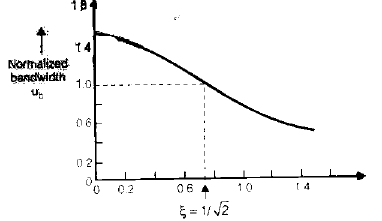• The denormalized bandwidth from equation (8.6) is given by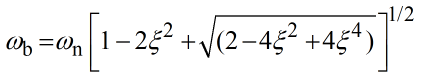• Let us now consider the step response of a second-order system. The expressions for the damped frequency of oscillation ωd and peak overshoot Mp of the step response for 0 ≤ ξ ≤ 1 are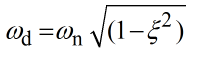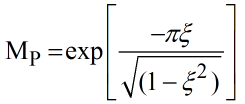• Mr, the resonant peak of the frequency response, and Mp, the peak overshoot of the step response for a second-order system, are plotted in the figure below for the various values. of x.
• The comparison of Mr and Mp plots shows that for 0 < ξ < 1/√2, the two performance indices are correlated as both are functions of the system damping factor x only. it means that a system with a given value of Mr of its frequency response must exhibit a corresponding value of Mp if subjected to a step input.
• For ξ > 1/√2, the resonant peak Mr does not exist and the correlation breaks down. This is not a serious problem as for this range of x, the step response oscillations are well damped and Mp is hardly perceptible.
• Similarly, the comparison of equations (8.4) and (8.8) reveals that there exists a definite correlation between the resonant frequency ωr of frequency response and damped frequency of oscillations of the step response. The ratio of these two frequencies is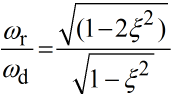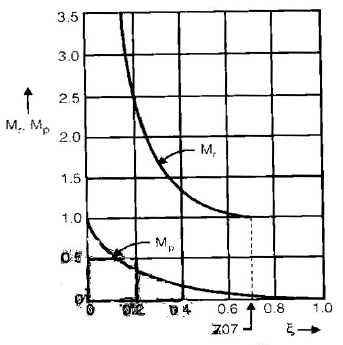Which is also a function of ξ and is plotted in the figure below.

• It is further observed from equations (8.7) that the bandwidth, a frequency domain concept, is indicative of the undamped natural frequency of a system for a given ξ and therefore indicative of the speed of response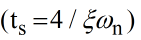, a time-domain concept.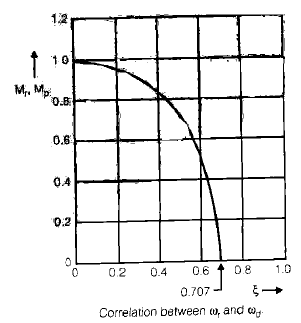Graphical Methods of Analysis of Frequency Domain

Polar Plots 

• Polar coordinates ( |GH| , ∠GH)

Let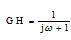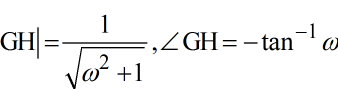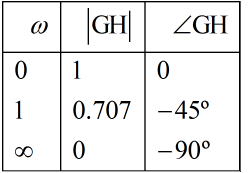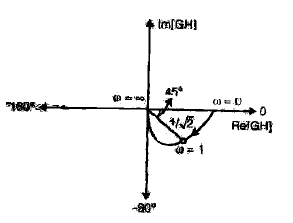(i) If G(s) H(s) =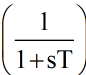G(jω) H(jω) =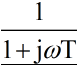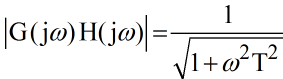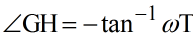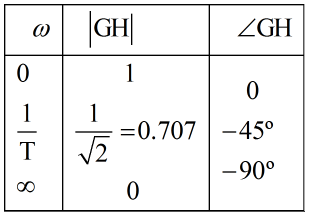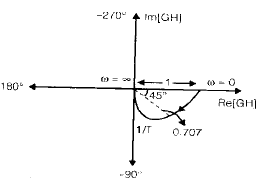(ii) If G(s) H(s)   = 1/s(1 + st)

G(jω) H(jω)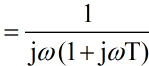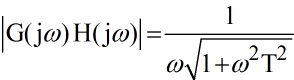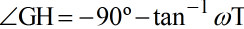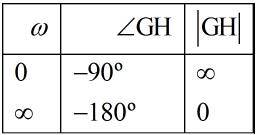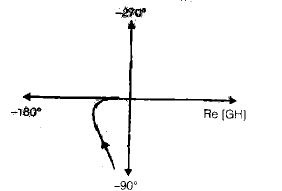• When a pole at origin is added, the head and tail of the polar plot shift by 90º in the clockwise direction.

(iii) If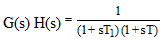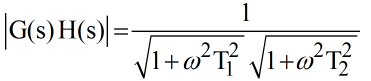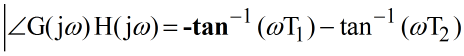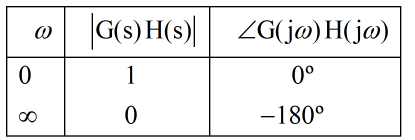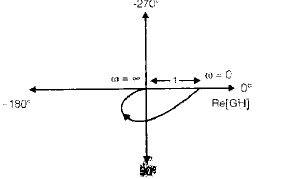Let, –tan-1 (ωT1) – tan-1 (ωT2) = - 90º

tan-1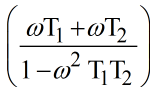= 900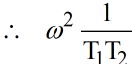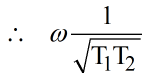When a pole on the real axis is added, the head of the polar plot shifts by 90º in the clockwise direction, and the tail remains the same.

(iv) If G(s) H(s) =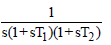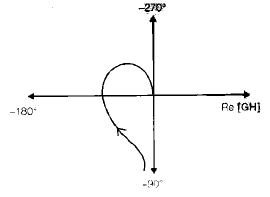(v) if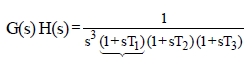Shift of Head → 5×90º = 450º 

Tail → 3×90º = 270º → due to poles at origin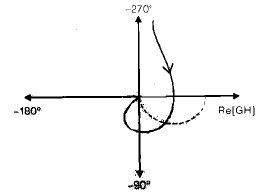(vi) If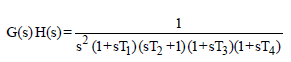Head → 5 × 90º =450º

Tail → 2×90º = 180º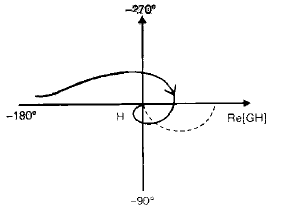POLAR PLOTS OF SIMPLE TRANSFER FUNCTION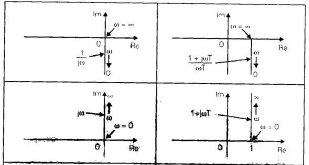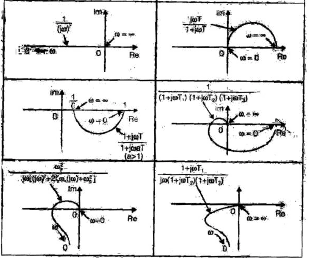Nyquist Plots 

Nyquist stability criterion [For a special case where G(s) H(s) has neither poles nor zeros on the jω = axis]; in the system shown in the figure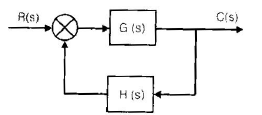If the open-loop transfer function G(s) H(s) has K poles in the right-half of s-plane and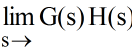= constant, then for stability, the G(jω) H(jω) locus, as w varies from – ∞ to ∞, must encircle the –1 + j0 point k times in the counterclockwise direction.

Remarks on the Nyquist Stability Criterion 
• This criterion can be expressed as

Z = N + P  or N = Z – P

where Z = Number of zeros of 1 + G(s) H(s) in the right. Half's plane

N = number of zeros of 1 + G(s) H(s) in the right-half s - plane

P = number of poles of G(s) H(s) in the right-half s-plane 

• If P is not zero, for a stable control system, we must have Z= 0, or N =- P, which means that we must have P counterclockwise encirclements of the –1 + j0 point. 
• If G(s) H(s) does not have any poles in the right half s plane, then Z = N. Thus for stability there must be no encirclement of the –1 + j0 point by the G(jω) H(jω) locus. In this case, it is not necessary to consider the locus for the entire jω=axis, only for the positive frequency portion.  The stability of such a system can be determined by seeing if the –1 + j0 point is enclosed by the Nyquist plot of G(jω). The region enclosed by the Nyquist plot is shown in the figure. For stability, the –1 + j0 point must lie outside the shaded region.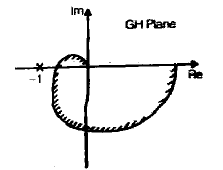• We must be careful when testing the stability of multiple loop systems since they may include poles in the right half s plane. (Note that although an inner loop may be unstable the entire closed-loop system can be made stable by proper design)
• Simple inspection of encirclements of the  (–1 + j0) point by the G(jω) H(jω) locus is not sufficient to detect instability in multiple-loop systems. In such cases, however, whether or not any pole of 1 + G(s) H(s) is in the right-half s plane can be determined easily by applying the Routh stability criterion to the denominator of G(s) H(s).
Stability Analysis
• If the Nyquist path in the s plane encircles Z zeros and P poles of [1 + G(s) H(s)] and does not pass through any poles or zeros of [1+ G(s) H(s)] as a representative point s moves in the clockwise direction along the Nyquist path, then the corresponding contour in the G(s) H(s) plane encircles the (–1 + j0) point N = Z – P times in the clockwise direction. (Negative values of N imply counterclockwise encirclements).
• In examining the stability of the linear control system using the Nyquist stability criterion, we see that three possibilities can occur.

1. There is no encirclement of the (– + j0) point. This implies that the system is stable if there are no poles of G(s)H(s) in the right half s-plane; otherwise, the system is unstable.

2. There is a counterclockwise encirclement or encirclements of the –1 +j0 point. In this case, the system is stable if the number of counterclockwise encirclements is the same as the number of poles of G(s)H(s) in the right-half a plane; otherwise, the system is unstable.

3. There is a clockwise encirclement or encirclements of the –1 +j0 point. In this case, the system is unstable.

NOTE:
Encirclement: Used in Nyquist stability criteria. It is for a closed path around (–1 + j0)

Enclosurement: Used in a polar plot. A point in the GH plane is said to be enclosed by the polar plot if it lies on the right-hand side of the plot when moving along the plot in the increasing direction of frequency from 0 to ∞ .

Phase Margin and Gain Margin
• The figure shows the polar plots of G(jω) for three different values of the open-loop gain K. For a large value of the gain K, the system is unstable. As the gain is decreased to a certain value, the G(jω) locus passes through the – 1 –j0 point.
• This means that with this gain value the system is on the verge of instability, and the system is on the verge of instability, and the system will exhibit sustained oscillations. For a small value of the gain K, the system is stable. In general, the closer the G(jω) locus comes to encircling the –1 + j0 point, the more oscillatory is the system response. The closeness of the G(jω) locus to the –1 + j0 point can be used as a measure of the margin of stability.
• This does not apply, however, to conditionally stable systems.
• It is common practice to represent the closeness in terms of phase margin and gain margin.
Phase Margin
• The phase margin is the amount of additional phase lag at the gain crossover frequency required to bring the system to the verge of instability.
• The gain crossover frequency is the frequency at which |G( jw)|, the magnitude of the open-loop transfer function is unity.
• The phase margin g is 180º plus the phase angle f of the open-loop transfer function at the gain crossover frequency, or g = 180º + f.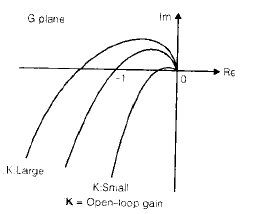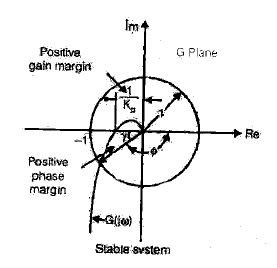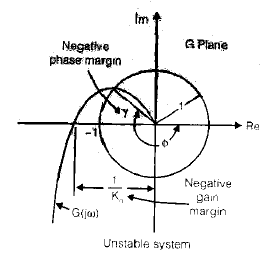• In the above figure of the polar plot, a line may be drawn from the origin to the point at which the unit circle crosses the G(jω) locus.
• The angle from the negative real axis to his line is the phase margin. The phase margin is positive for g > 0 and negative for g < 0.  For the minimum phase system to be stable, the phase margin must be positive.
• In the logarithmic plots, the critical point in the complex plane corresponds to the 0 dB and – 180º lines.
Gain Margin
• The gain margin is the reciprocal of the magnitude |G(jω)| at the frequency where the phase angle is – 180º.
• Defining the phase crossover frequency ω1 to be the frequency at which the phase angle of the open-loop transfer function equals –180º gives the gain margin Kg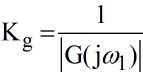in terms of decibels,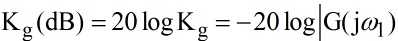• The gain margin expressed in decibels is positive if Kg is greater than unity and negative if Kg is smaller than unity.
• A positive gain margin (in decibels) means that the system is stable, and a negative gain margin (in decibels) means that the system is unstable.  The gain margin and phase margins for different plots have been shown in Figures (a), (b), and (c).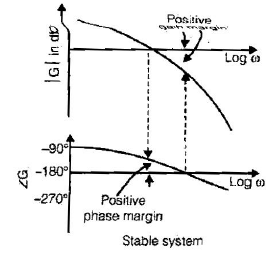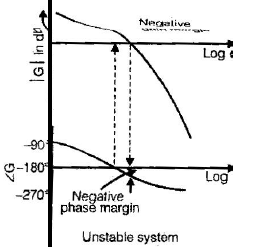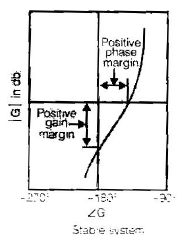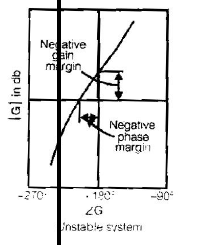(phase and gain margins of stable and unstable systems)

(a) Bode diagrams; (b) polar Plots; (c) log-magnitude versus phase plots.

• For a stable minimum phase system (defined in the last section of this chapter), the gain margin indicates how much the gain can be increased before the system becomes unstable.
• For an unstable system, the gain margin is indicative of how much the gain must be decreased to make the system stable.
• The gain margin of a first or second-order system is infinite since the polar plots for such systems do not cross the negative real axis. Thus, theoretically, first or second-order systems cannot be unstable.

NOTE:

• However, that so-called first or second-order systems are only approximations in the sense that small-time lags are neglected in deriving the system equations and are thus not truly first or second-order systems.
• If these small lags are accounted for, the so-called first or second-order systems may become unstable. It is important to point out that for a nonminimum phase system the stability condition will not be satisfied unless the G(jω) plot encircles the (–1 + j0) point. Hence, a stable non-minimum phase system will have a negative phase and gain margin.
• It is also important to point out that conditionally stable systems will have two or more phase crossover frequencies, and some higher-order systems with complicated numerator dynamics may also have two or more gain crossover frequencies, as shown in Figure.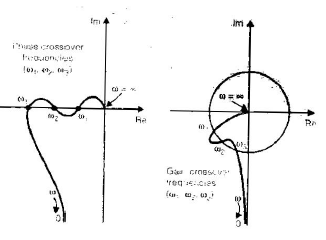• For stable systems having two or more gain crossover frequencies, the phase margin is measured at the highest gain crossover frequency.

A Few Comments on phase and Gain Margins

• The phase and gain margins of a control system are a measure of the closeness of the polar plot to the (–1 + j0) point. Therefore, these margins may be used as design criteria.
• It should be noted that either the gain margin alone or the phase margin alone does not give a sufficient indication of the relative stability. Both should be given in the determination of relative stability.
• For a minimum phase system, both the phase and gain margins must be positive for the system to be stable. Negative margins indicate instability.
• Proper phase and gain margins ensure us against variations in the system components and are specified for definite values of frequency. The two values bound the behavior of the closed-loop system near the resonant frequency.
• For satisfactory performance, the phase margin should be between 30º and 60º, and the gain margin should be greater than 6 dB. with these values, a minimum phase system has guaranteed stability, even if the open-loop gain and time constants of the components vary to a certain extent. Although the phase and gain margins are given only rough estimates of the effective damping ratio of the closed-loop system, they do offer a convenient means for designing control systems or adjusting the gain constant of systems.

Closed-Loop Frequency Response
• Let us define the magnitude of the closed-loop frequency response as M and the phase angle as α, or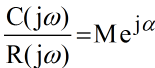• In the following, we shall find the constant magnitude loci and constant phase-angle loci. such loci are convenient in determining the closed-loop frequency response from the polar plot or Nyquist plot.

Constant magnitude Loci (M-circles) 

• to obtain the constant magnitude loci, let us first note that G(jω) is a complex quantity and can be written as follows: G(jω) = X + jY where X and Y are real quantities. Then M is given by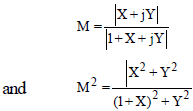Hence, X2 (1–M2)–2M2 X–M2 + (1 – M2)Y2 =0

• If M = 1, then from the above equation (8.10) we obtain X =–1/2. This is the equations of a straight line parallel to the Y-axis and passing through the point (–1/2, 0).
• If M ≠ 1, the equation can be written as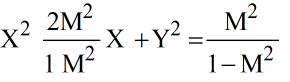if the term [M2/(1–M2)]2 is added to both sides of this last equation, we obtain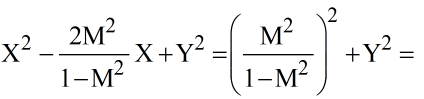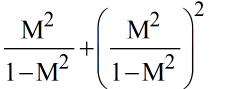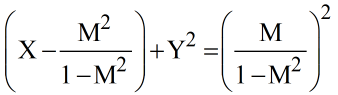• Equation (8.11) is the equation of a circle with centre at X = +M2/(M2–1), Y=0 and with radius |M / (M2 - 1)| .
• The constant M loci on the G(s) plane are thus a family of circles. The centre and radius of the circle for a given value of M can be easily calculated
• For M>1, the centers of the M circles lie to the left of the (–1 +j0) point. Similarly, as M becomes smaller compared with 1, the M circle becomes smaller and converges to the origin. For 0< M <1, the centers of the M circles lie to the right of the origin.
• M = 1 corresponds to the locus of points equidistant from the origin and from the (-1 + j0) point. As stated earlier, it is a straight line passing through the point (-1/2, 0) and parallel to the imaginary axis.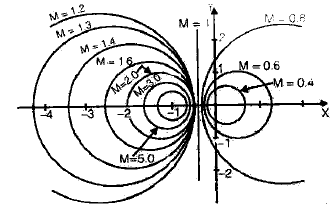• The M circles are symmetrical with respect to the straight line corresponding to M =1 and with respect to the real axis.

Constant Phase-angle Loci (N-circles)

• We shall obtain the phase angle α in terms of X and Y. Since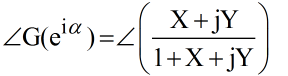the phase angle α is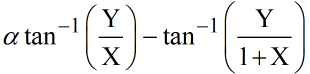if we define, tanα = N then,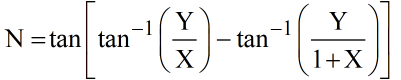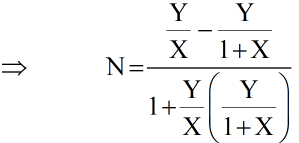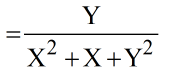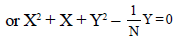The addition of (1/4) + 1/(2N)2 to both sides of this last equation yields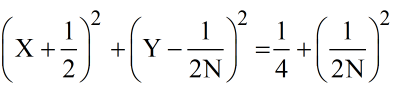This is an equation of a circle with center at X = –1/2, Y = 1/(2N), and with a radius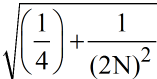Since the equation is satisfied when X=Y=0 and X=–1, Y = 0 regardless of the value of N, each circle passes through the origin and the (–1 + j0) point. The constant N loci can be drawn easily once the value of N is given. A family of constant N circles is shown in the figure below with a as a parameter.

It should be noted that the constant N locus for a given value of a is actually not the entire circle but only an arc. In other words, the α = 30º and α = 150º arcs are parts of the same circle. This is so because the tangent of an angle remains the same if ± 180º (or multiples of it) is added to the angle.

The use of the M and N circles enables us to find the entire closed-loop frequency response from the open-loop frequency response G(jω) without calculation the magnitude and phase of the closed-loop transfer function at each frequency. The intersections of the G(jω) locus and the M circles and N circles given the values of M and N at frequency points on the G(jω) locus. The N circles are multivalued in the sense that the circle for α = α1 and that for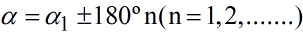are the same. In using the N circles for the determination of the phase angle of closed-loop systems, we must interpret the proper value of a. to avoid any error, start at zero frequency, which corresponds to α = 0º, and proceed to higher frequencies. The phase-angle curve must be continuous.

• Graphically, the intersection of the G(jω) locus and M circles give the values of M at the frequencies denoted on the G(jω) locus. Thus, the constant M circle with the smallest radius that is tangent to the G(jω) locus gives the value of the resonant peak magnitude Mr. If it is desired to keep the resonant peak value less than a certain value, then the system should not enclose the critical point (–1 + j0 point) and at the same time, there should be no intersections with the particular M circle and the G(jω) locus.
• Figure (a) shows the G(jω) locus superimposed on a family of M circles. Figure (b) shows the G(jω) locus superimposed on the family of N circles. From these plots, it is possible to obtain the closed-loop frequency response by inspection. it is seen that the M = 1.1 circle intersects the G(jω) locus at frequency point ω = ω1. This means that at this frequency the magnitude of the closed-loop transfer function is 1.1.
• In figure (a), the M = 2 circle is just tangent to the G(jω) locus. Thus, there is only one point on the G(jω) locus for which |C(jω) / R (jω)| is equal to 2. Figure (c) in the next page shows the closed-loop frequency- response curve for the system. The upper curve is the M versus frequency w curve and the lower curve is the phase angle a versus frequency ω curve.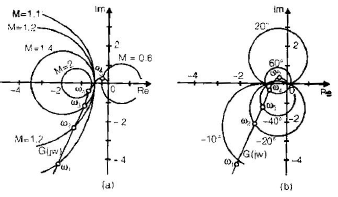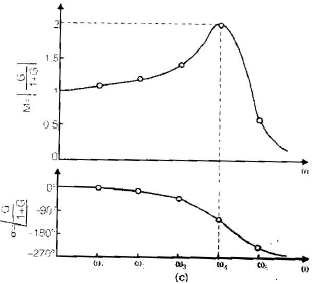• The resonant peak value is the value of M corresponding to the M circle of the smallest radius that is tangent to the G(jω) locus. Thus, in the Nyquist diagram, the resonant peak value Mr and the resonant frequency wr can be found from the M-circle tangency to the G(jω) locus. (In the present example, Mr = 2 and ωr  = ω4).

General Procedure for Plotting Bode-Diagrams

• First, rewrite the sinusoidal transfer function G(jω) H(jω) as a product of basic factors discussed above.
• Then identify the corner frequencies associated with these basic factors.
• Finally, draw the asymptotic log-magnitude curves with proper slopes between the corner frequencies. The exact curve, which lies close to the asymptotic curve, can be obtained by adding proper corrections.
• The phase-angle curve of G(jω) H(jω) can be drawn by adding the phase-angle curves of individual factors.

NOTE: The use of Bode diagrams employing asymptotic approximations requires much less time than other methods that may be used for computing the frequency response of a transfer function. The ease of plotting the frequency-response curves for a given transfer function and the ease of modification of the frequency-response curve as compensation is added are the main reasons why Bode diagrams are very frequently used in practice.

Offer running on EduRev: Apply code STAYHOME200 to get INR 200 off on our premium plan EduRev Infinity!

65 docs|37 tests

,

,

,

,

,

,

,

,

,

,

,

,

,

,

,

,

,

,

,

,

,

,

,

,

,

,

,

;